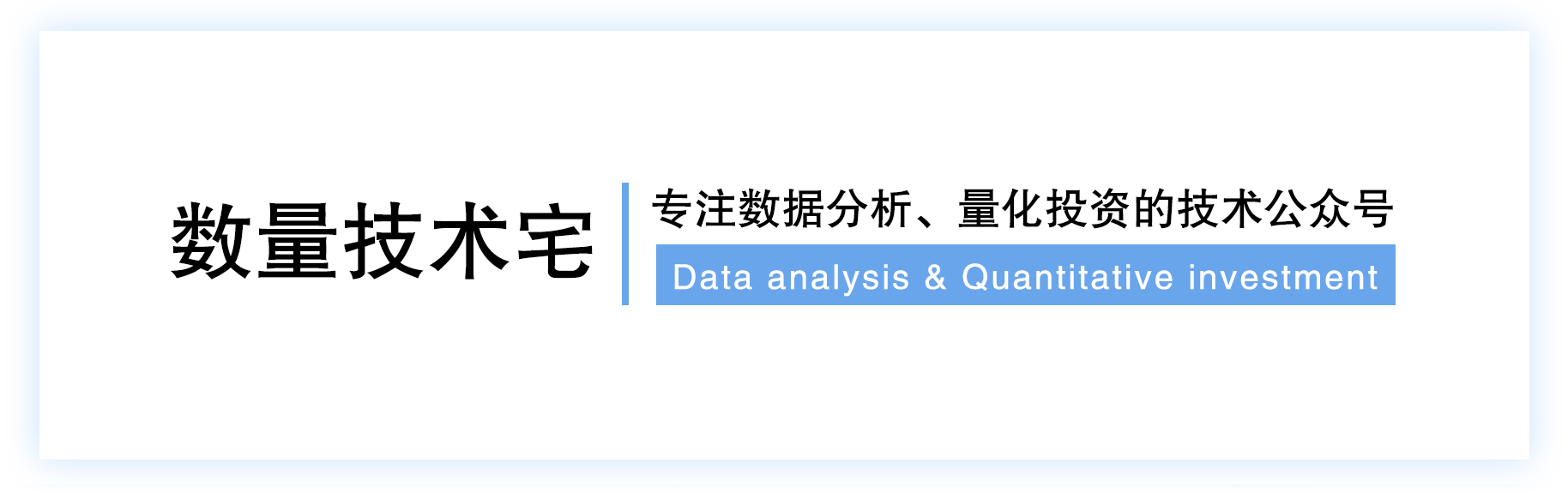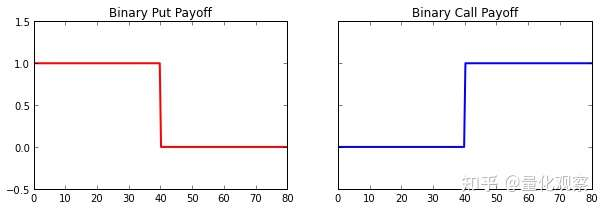### 扩展期权定价模型到二元期权定价## 欧式期权定价回顾

while i < num_iterations:
S_T = generate_asset_price()
payoffs += payoff_function(S_T)
i += 1

option_price = exp(-r*T) * (payoffs / num_iterations)

## 二元期权def binary_call_payoff(K, S_T):
if S_T >= K:
return 1.0
else:
return 0.0

## 模拟计算过程

def gbm(S, v, r, T):
return S * exp((r - 0.5 * v**2) * T + v * sqrt(T) * random.gauss(0,1.0))

import random
from math import exp, sqrt

def gbm(S, v, r, T):
return S * exp((r - 0.5 * v**2) * T + v * sqrt(T) * random.gauss(0,1.0))

def binary_call_payoff(K, S_T):
if S_T >= K:
return 1.0
else:
return 0.0

# parameters
S = 40.0 # asset price
v = 0.2 # vol of 20%
r = 0.01 # rate of 1%
maturity = 0.5
K = 40.0 # ATM strike
simulations = 50000
payoffs = 0.0

# run simultaion
for i in xrange(simulations):
S_T = gbm(S, v, r, maturity)
payoffs += binary_call_payoff(K, S_T)

# find prices
option_price = exp(-r * maturity) * (payoffs / float(simulations))

print 'Price: %.8f' % option_price

## 检验结果>>> from scipy.stats import norm
>>> from math import exp, log, sqrt
>>> S, K, v, r, T = 100.0, 100.0, 0.2, 0.01, 0.5
>>> d2 = (log(S/K) + (r - 0.5 * v**2) * T) / v*sqrt(T)
>>> print exp(-r * T) * norm.cdf(d2)
0.490489409105## 往期干货分享推荐阅读

Omega System Trading and Development Club内部分享策略Easylanguage源码

AMA指标原作者Perry Kaufman 100+套交易策略源码分享

【数量技术宅|金融数据分析系列分享】为什么中证500（IC）是最适合长期做多的指数

【数量技术宅|金融数据分析系列分享】如何正确抄底商品期货、大宗商品

【数量技术宅|量化投资策略系列分享】股指期货IF分钟波动率统计策略

【数量技术宅 | Python爬虫系列分享】实时监控股市重大公告的Python爬虫